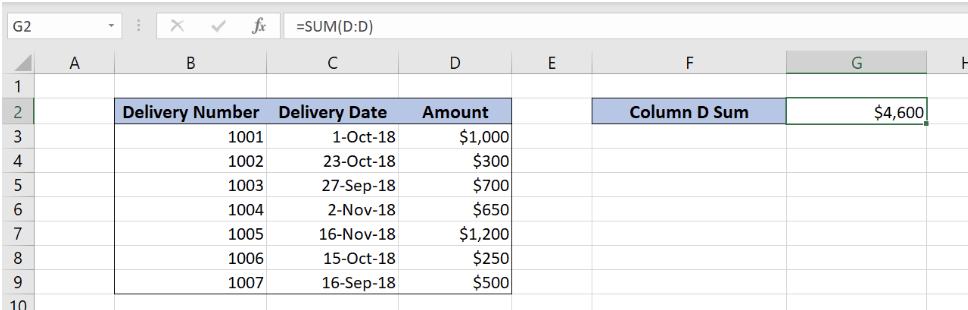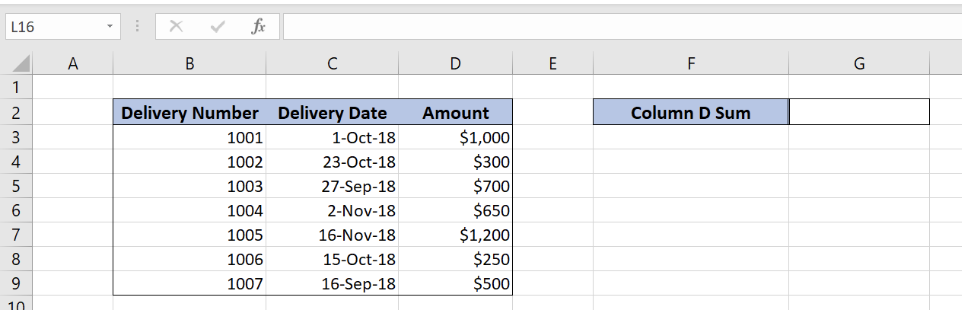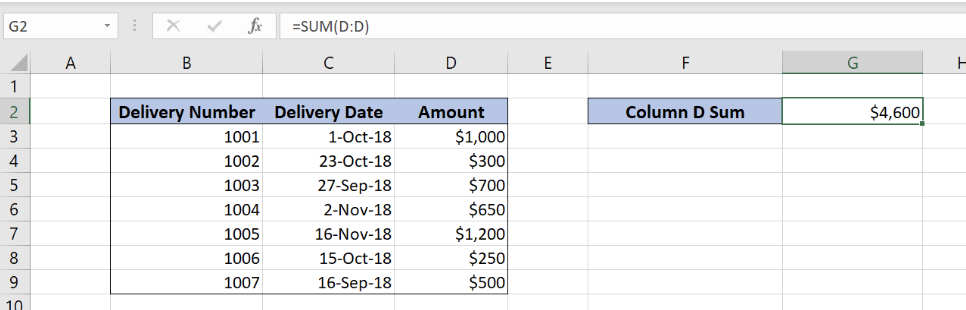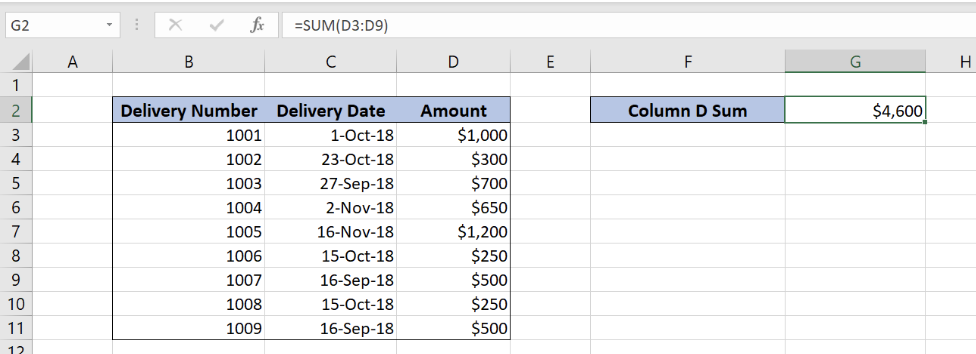Get instant live expert help with Excel or Google Sheets“My Excelchat expert helped me in less than 20 minutes, saving me what would have been 5 hours of work!”

#### Post your problem and you’ll get Expert help in seconds.

Your message must be at least 40 characters
Our professional Expert are available now. Your privacy is guaranteed.

# Sum entire column

Excel allows us to sum all values from any column by using the SUM function. This step by step tutorial will assist all levels of Excel users in summing values from a column.Figure 1. The final result of the SUM function

## Syntax of the SUM Formula

The generic formula for summing entire column looks like:

`=SUM(range)`

The parameter of the SUMIF function is:

• range – can be a range of cells or an entire column

## Setting up Our Data for the SUM Function

Our table consists of 3 columns: “Delivery Number” (column B), “Delivery Date” (column C) and “Amount” (column D). In cell G2, we want to get the sum of all the Amounts (entire column D).Figure 2. Data that we will use in the SUM example

## Sum Entire Column Using the SUM Function

We want to sum all amounts from column D in the cell G2. The formula looks like:

`=SUM(D:D)`

The range parameter is D:D, as we want to sum the entire column D.

To apply the SUM function, we need to follow these steps:

• Select cell G2 and click on it
• Insert the formula: `=SUM(D:D)`
• Press enterFigure 3. Using the SUM function to sum the entire column

We see in this example that the formula sums all the amounts from the column D. The sum in the cell G2 is \$4.600.

In this example, we can also put D3:D9 as the range of the SUM function. The result would be the same as in the previous example. However, if we add new rows in the table, the sum will stay the same, as our range is fixed on D3:D9. Let’s look at the example:Figure 4. Using the SUM function with the fixed range

We added two now rows in the data table. As you can see in the picture, the result of the SUM function in the cell G2 remained the same.

## Notes

We must have in mind that the SUM function sums all the rows, even the hidden ones. So, in order to check the result of the function, we need to unhide all the rows.

Most of the time, the problem you will need to solve will be more complex than a simple application of a formula or function. If you want to save hours of research and frustration, try our live Excelchat service! Our Excel Experts are available 24/7 to answer any Excel question you may have. We guarantee a connection within 30 seconds and a customized solution within 20 minutes.

### Did this post not answer your question? Get a solution from connecting with the expert.Another blog reader asked this question today on Excelchat:
Solution examplesI am looking for a formula to SUM column L, if Column A matched the relevant date and column C matches the name
Solved by Z. Y. in 16 minsHi, I'm making myself a weekly budget spreadsheet. I've made a sheet with all my monthly outgoings, and I've worked out how to use SUMIF to combine the amounts for each day, but I want to include a reference and don't know how to combine text cells based on a specific date reference... does that make sense?
Solved by E. U. in 60 minsI need a formula to pop at the upper left corner of a spreadsheet. If I enter the month "January," I want the column number sum of January =SUM(AB11:AB75) from another section on the same excel page to pop right below the "January" cell, and not display the formula expression, but see the \$100.
Solved by T. Q. in 40 minsCan't add (SUM) in imported numbers from bank account
Solved by F. C. in 40 minsI need a formula to combine D2 to D100 to add together a column of numbers, then take away the same amount on the same row when column E is filled. i.e. column D is a price of an item, so the formula must calculate the total, then when the item is sold an 'a' is marked next to the item in column E, the formula then must deduct this amount from the total
Solved by X. W. in 20 mins## Subscribe to Excelchat.coAnother blog reader asked this question today on Excelchat: